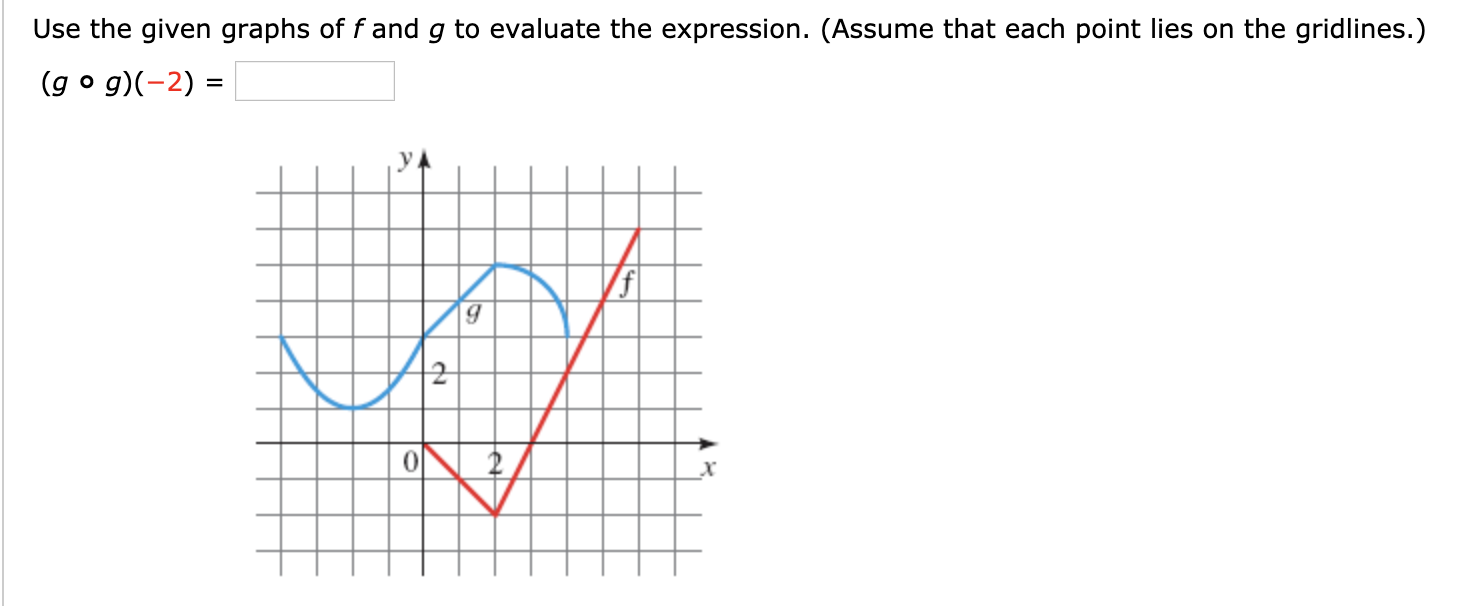# Use the given graphs of fand g to evaluate the expression. (Assume that each point lies on the gridlines.)(g o g)-2)=22

Question
1 viewshelp_outlineImage TranscriptioncloseUse the given graphs of fand g to evaluate the expression. (Assume that each point lies on the gridlines.) (g o g)-2) = 2 2 fullscreen
check_circle

Step 1

Given that a graph of function f & g.

We need to evaluate the expression:

Step 2

General  formula for function composition is:

Step 3

Here given t...

### Want to see the full answer?

See Solution

#### Want to see this answer and more?

Solutions are written by subject experts who are available 24/7. Questions are typically answered within 1 hour.*

See Solution
*Response times may vary by subject and question.
Tagged in

### Calculus